• ### 3D Convex Collision Detection, Part 2: EPA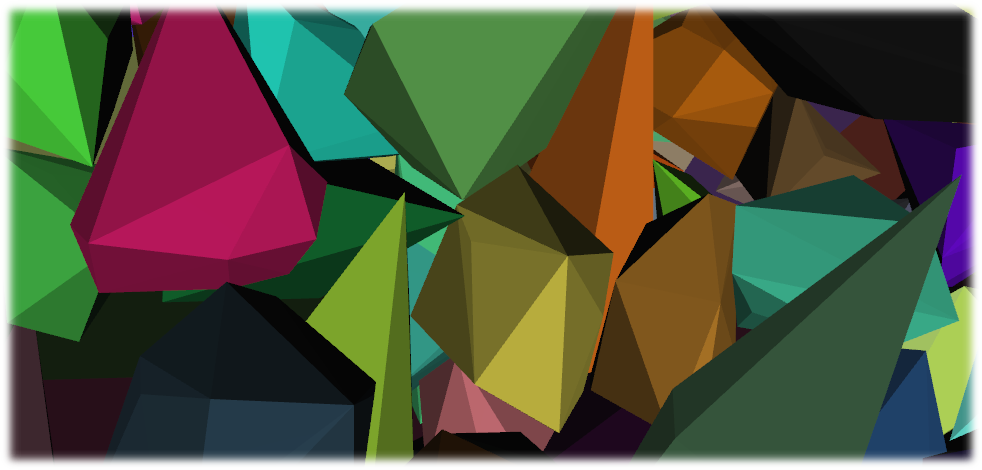This is the second of a three article series that will teach you how to efficiently detect collisions, generate contact information, and generate convex hulls for 3D objects. This article focuses on generating contact information using the Expanding Polytope Algorithm (EPA). It is highly recommended that you read my previous article on GJK intersection testing if you have not already.

# EPA:

EPA is the perfect complement to the GJK intersection algorithm, as it relies on the same support mappings and requires a polytope containing the origin, which is exactly what GJK has built for us. EPA uses this polytope to iteratively search for closest point on the hull of the Minkowski difference to the origin. Once we have this point we can use it to extrapolate the collision normal and penetration depth. To this end we need to make a single small modification to our GJK implementation.

To extrapolate the contact point we are going to need the individual support map point for one of the colliders in addition to each combined point. The easiest way to do this would be to just replace all instances of your Vector3 class with a struct that holds both the combined point and the component point of one of the shapes:

```struct SupportPoint {
HDXVector3 v; // the minkowski difference point

// the individual support points
HDXVector3 sup_a;
HDXVector3 sup_b; // not actually necessary

BOOL operator==(const SupportPoint &r) const { return v == r.v; }
};

auto lam_support = [&](HDXVector3 d)->SupportPoint {
d.setNormal(); // to quell numerical instability
SupportPoint ret;
ret.sup_a = bv0->getFurthestPointInDirection(d);
ret.sup_b = bv1->getFurthestPointInDirection(-d);
ret.v = ret.sup_a - ret.sup_b;
return ret;
};
```

# Data structures:

Just like in the GJK algorithm we will need a way to cleanly keep track of our polytope. To this end I keep a list of simple triangle structs that hold the three component points and the precomputed normal. Later on we will also need an edge struct that holds two points.

```struct Triangle {
SupportPoint points;
HDXVector3 n;

Triangle(const SupportPoint &a,const SupportPoint &b,const SupportPoint &c) {
points = a;
points = b;
points = c;
n = ((b.v-a.v) % (c.v-a.v)).getNormal();
}
};

struct Edge {
SupportPoint points;

Edge(const SupportPoint &a,const SupportPoint &b) {
points = a;
points = b;
}
};

// list because we will be removing and adding a lot
std::list<Triangle> lst_triangles;
std::list<Edge> lst_edges;
```

## Here is the basic structure for the algorithm:

1: Build the initial polytope from the tetrahedron produced by GJK.
2: Pick the closest face of the polytope to the origin.
3: Generate the next point to be included in the polytope by getting the support point in the direction of the picked triangle’s normal.
4: If this point is no further from the origin than the picked triangle (by a reasonable threshold), then go to 7
5: Remove all faces from the polytope that can be seen by this new point, this will create a hole that must be filled with new faces built from the new support point and the remaining points from the old faces.
6: Go to 2.
7: Use the current closest triangle to the origin to extrapolate the contact information.

# Building the initial polytope:

The initial polytope consists of the four triangles that made up the simplex that GJK terminated with.

```// add the GJK simplex triangles to the list
lst_triangles.emplace_back(sim.a,sim.b,sim.c);
lst_triangles.emplace_back(sim.a,sim.c,sim.d);
lst_triangles.emplace_back(sim.a,sim.d,sim.b);
lst_triangles.emplace_back(sim.b,sim.d,sim.c);
```

# Finding the closest face to the origin:

Testing the distance from a triangle to the origin is easy, all we need to do is take the dot product of the triangle’s normal with one of the triangle’s points. Once we have the closest face we need to generate the support point furthest in the direction of the closest face’s normal via the lam_support function/lambda.
Visual breakdown in 2D:
The 2D version of EPA should be easier to wrap your mind around. The concept is the same, however instead of dealing with triangles it deals with segments.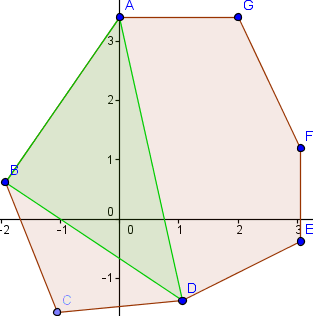The red shape is the Minkowski Difference of our colliders. The green triangle is the simplex created by GJK. We need to loop over all three segments and find the one that is closest to the origin. We do this by taking the dot product of the segment’s normal and one of its points. In this case the closest segment is BD.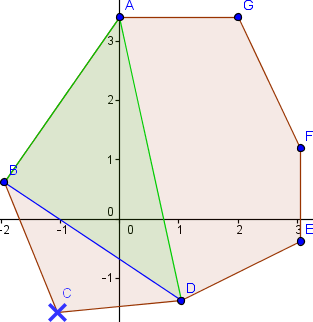Now we call our support function and get the point furthest in the direction of the segment BD’s normal, C. We now must remove the segment BD and create new segments using the new point C, resulting in BC and CD.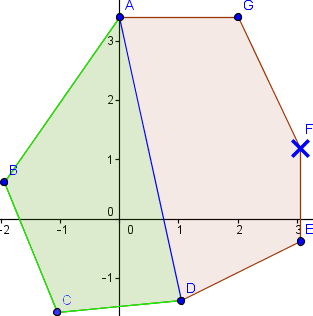Now we repeat the process, finding that the closest segment to the origin is AD, and the new support point in AD’s normal’s direction is F. We again remove the segment AD and add segments DF and FA.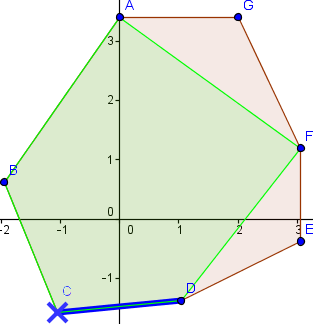We repeat the process one last time, finding that the closest segment is CD. Using our support function and CD’s normal we find that our next point is C. Since we did not move any further from the origin with our new point we are done and we can move onto the extrapolation portion of the algorithm.

# Adding the new point and updating the polytope:

Once we add a new point to the polytope we need to remove all of the triangles that can be seen by this point. This is accomplished by a simple winding check, the same used in graphics culling algorithms.

```if(normal * point – (normal * pointOnTriangle) > 0)
// or more concisely
if(normal * (point – pointOnTriangle)> 0)
```

Now that we can identify which triangles must be removed we are faced with the question of how we are going to deal with filling in the hole that their removal creates. To do this we need some way of identifying which edges form the hole. This can be calculated by keeping a specialized list of edges that have been removed as we iterate over the triangles. The trick is that if two triangles that are removed share an edge then that edge is gone for good, but if an edge is used by only one removed triangle then it will form part of the hole. Shown here if we remove only triangle ACB all three edges AC, BC, and BA will remain as they were only shared by the one triangle.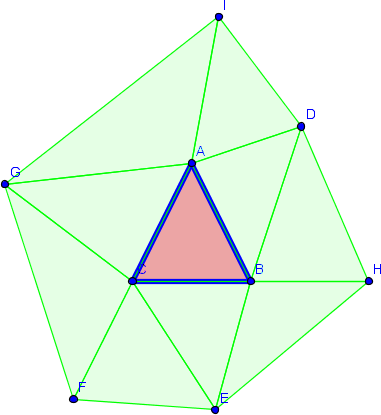But if we were to also remove triangle ABD then the edge formed with points A and B would not remain, as it was shared by both triangles.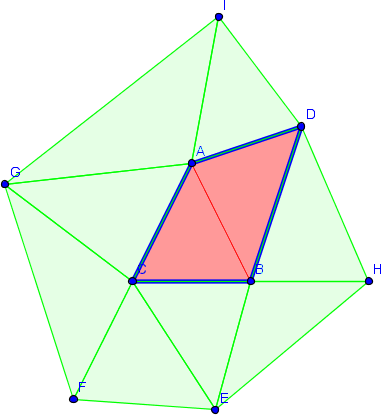It is quite easy to maintain a list of valid edges by adding all three edges of every removed triangle to the list with the following rule: In the event that an edge is added that already exists with the same points in the opposite order the old edge is removed and the new edge is not added. Like so:

```// process the specified edge, if another edge with the same points in the
// opposite order exists then it is removed and the new point is also not added
// this ensures only the outermost ring edges of a cluster of traingles remain
// in the list
auto lam_addEdge = [&](const SupportPoint &a,const SupportPoint &b)->void {
for(auto it = lst_edges.begin(); it != lst_edges.end(); it++) {
if(it->points==b && it->points==a) {
//opposite edge found, remove it and do not add new one
lst_edges.erase(it);
return;
}
}
lst_edges.emplace_back(a,b);
};

for(auto it = lst_triangles.begin(); it != lst_triangles.end();) {
//can this face be 'seen' by entry_cur_support?
if(it->n * (entry_cur_support.v - it->points.v) > 0) {
it = lst_triangles.erase(it);
continue;
}
it++;
}
```

Here is an illustration of the concept in 3D, imagine that the point to be added is out in front of the screen.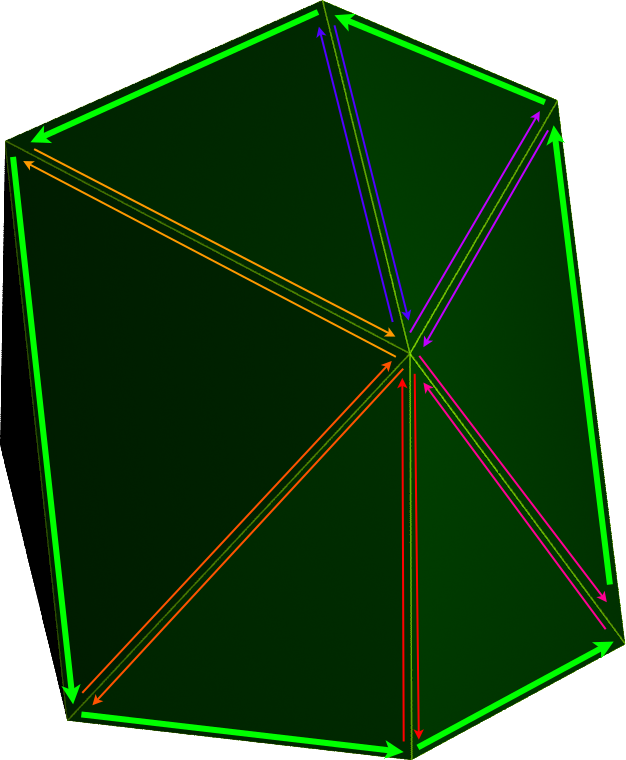As we can see all of the triangles are wound counter-clockwise, this means that the points of shared edges are given in the opposite order for each triangle. The above method would leave us with only the edges shown in bold green, along the rim of the shape.
Now that we have the valid edge list it is trivial to fill in the hole, we just need to iterate over the edge list and create new triangles using the new support point as A and each edge’s points as B and C.

```// create new triangles from the edges in the edge list
for(auto it = lst_edges.begin(); it != lst_edges.end(); it++) {
lst_triangles.emplace_back(entry_cur_support,it->points,it->points);
}

lst_edges.clear();
```

# Extrapolating the contact information:

Now for the fun part. Once we have found a triangle that is “close enough” to the hull of the Minkowsi Difference we can project the origin onto it and calculate the barycentric coordinates of this point with respect to the triangle. These barycentric coordinates allow us to transform from “Minkowski” space to world space by linearly combining them with the individual support points of the triangle (the individual support points are described near the top of the article). That will give us our contact point. The contact normal is just the negative normal of the closest triangle, and the penetration depth is the distance from the closest triangle to the origin, which we have already calculated.
First we need a function to calculate the barycentric coordinates, I got this function from Crister Erickson’s Real-Time Collision Detection.

```void barycentric(const HDXVector3 &p,const HDXVector3 &a,const HDXVector3 &b,const HDXVector3 &c,float *u,float *v,float *w) {
// code from Crister Erickson's Real-Time Collision Detection
HDXVector3 v0 = b - a,v1 = c - a,v2 = p - a;
float d00 = v0*v0;
float d01 = v0*v1;
float d11 = v1*v1;
float d20 = v2*v0;
float d21 = v2*v1;
float denom = d00 * d11 - d01 * d01;
*v = (d11 * d20 - d01 * d21) / denom;
*w = (d00 * d21 - d01 * d20) / denom;
*u = 1.0f - *v - *w;
}
```

## Generate the contact information:

```// i am storing an iterator to the closest triangle to the origin
// rather than copying it for efficiency (entry_cur_triangle_it)
// entry_cur_support is the new support point in the direction of
// the current triangle's normal, _EXIT_THRESHOLD is needed for shapes
// with infinite points like a sphere, as we will never find the absolute
// furthest triangle, i use 0.0001f for it

// checks how much further this new point will takes us from the origin
// if it is not far enough then we assume we have found the closest triangle
// on the hull from the origin
if((entry_cur_triangle_it->n*entry_cur_support.v - entry_cur_dst < _EXIT_THRESHOLD)) {
// calculate the barycentric coordinates of the closest triangle with respect to
// the projection of the origin onto the triangle
float bary_u,bary_v,bary_w;
barycentric(entry_cur_triangle_it->n * entry_cur_dst,
entry_cur_triangle_it->points.v,
entry_cur_triangle_it->points.v,
entry_cur_triangle_it->points.v,
&bary_u,
&bary_v,
&bary_w);

// collision point on object a in world space
HDXVector3 wcolpoint((bary_u*entry_cur_triangle_it->points.sup_a)+
(bary_v*entry_cur_triangle_it->points.sup_a)+
(bary_w*entry_cur_triangle_it->points.sup_a));

// collision normal
HDXVector3 wcolnormal = -entry_cur_triangle_it->n;

// penetration depth
float wpendepth = entry_cur_dst;
break;
}
```

# What now?:

Now we have a robust way of detecting intersections and extracting accurate contact information from them, which we can use for physics collision response. The main shortfall of this method is that it only works on convex objects, my next article will go over the quickhull algorithm which will allow you to build a convex hull around any set of points that you can use with GJK and EPA.

## FULL SOURCE CODE (integrated into an engine) IS AVAILABLE HEREE: Lattice3D Engine BitBucket

### 6 Responses to “3D Convex Collision Detection, Part 2: EPA”

1.Gregg Baugh says:

I have to tell you that it’s hard to find your articles in google, i
found this one on 11 spot, you should build some quality backlinks in order to
k2 seo tips

2.weight loss says:

3.Matthias says:

Hi thank your for this great article.

I have one question. Could it be possible that your GJk-EPA is not able to calculate the penetration depth if the input data are laying in one plane? For example my test data got the form (x,y,0). I think your Tetrahedon will be a degenerate one in the xy-plane.
In your EPA if u try to find the closet triangle and afterwards the penetration depth, each triangle will contains the origin and the pen depth will be always zero.
Isnt it the right way to reduce in this case the problem to 2D and calculate the distance between origin and the line segments of one triangle ?

4.nayglr says:

acheter levitra moins cher

5.child says:

ankara seks

6.aodcjpmblx says:

order cialis lyrica side effects in women cialis 10 mg